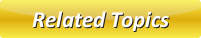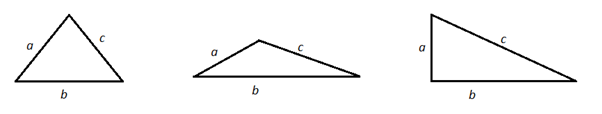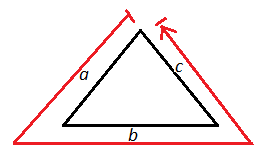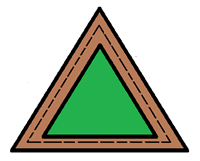# Perimeter of Triangle

A three sided polygon which has three vertices and three angles is called a triangle. Equilateral triangle, Isosceles triangle and right angled triangle are the three different types of triangles. ΔABC represents a triangle with vertices or corners A, B, and C and side lengths a, b and c.�### How to calculate the perimeter of a triangle?

A Perimeter of the triangle is the total distance along the outside of the triangle.Steps to calculate the Perimeter of a triangle:
• Note the lengths of all the sides of the triangle � � � � ��
• Add all the three lengths i.e, a+b+c
• Perimeter of a Triangle is given by a+b+c.

Note: The perimeter of an equilateral triangle is 3 times the length of each side as all the three sides are equal.

## Perimeter of a Triangle problems

Problem 1: A triangle sides measure 6 centimeters, 8 centimeters and 7 centimeters. What is its perimeter?

Solution: Length of  each side of triangle,

a = 6 cm

b = 8 cm

c = 7 cm

Perimeter of the Triangle = a+b+c=6+8+7= 21 cm.

Problem 2: Find the perimeter of an equilateral triangle with each side measuring 3 cm?

Solution: Length of  each side of an equilateral triangle, a = b = c = 3 cm

Perimeter of an equilateral triangle = 3*side length =3(3)=9

Thus the perimeter of given equilateral triangle is 9 cm.

Problem 3:  In a triangle two sides are 4 inches and 6 inches long. Its perimeter is 15 inches. Find the length of the third side.

Solution:  Given two sides of triangle, say a = 4 in and b = 6 in.

Let the third side be c inches.

The Perimeter of the triangle = 15 in. .......(1)

Also by the definition of Perimeter of triangle,

Perimeter of triangle = a+b+c=4+6+c  ...........(2)

Equating (1) and (2),

4+6+c=15

10+c=15

c=15-10 =5.

The third side is of length 5 inches.

Problem 4: Christy jogs around a triangular path which is equilateral in a park. If he jogs same distance every day and covers 36 kms in 6 days, what is the length of each side of the path?Solution: The distance jogged by Christy in 6 days = 36 kms.

The distance jogged by Christy in 1 days = 36/6 = 6 kms.

Perimeter of the equilateral triangle path = The distance covered in a day

3 x length of each side of the path = 6 kms

Length of each side of the path = 6/3  = 2 kms

Latest Articles

Average Acceleration Calculator

Average acceleration is the object's change in speed for a specific given time period. ...

Free Fall Calculator

When an object falls into the ground due to planet's own gravitational force is known a...

Permutation

In Mathematics, the permutation can be explained as the arrangement of objects in a particular order. It is an ordered...

Perimeter of Rectangle

A rectangle can be explained as a 4-sided quadrilateral which contains equal opposite sides. In a rectangle

Perimeter of Triangle

A three sided polygon which has three vertices and three angles is called a triangle. Equilateral triangle...